# BIT110 Business Mathematics and Statistics Assignment Solution## A. The frequency distribution of the examination score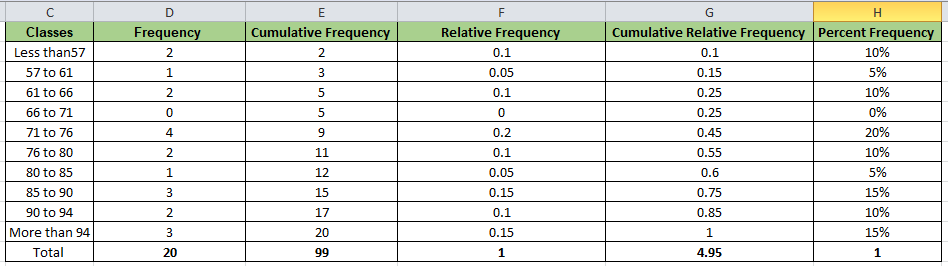B. Histogram (examination score)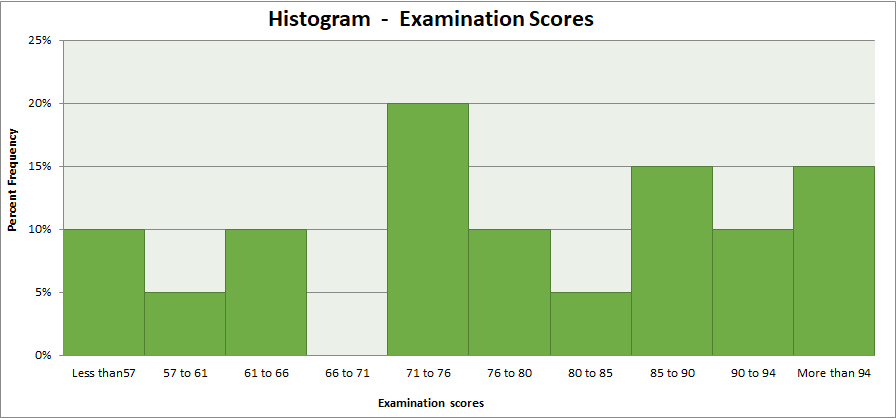1. Bell curve has not been observed
2. High variation has been observed
3.
Presence of negative skew

Based on the above underling factors, it can be concluded that examination scores does not show normal distribution (Harmon, 2016).

### Supply dependent variable represents as Y

Unit price independent variable represented as X.

###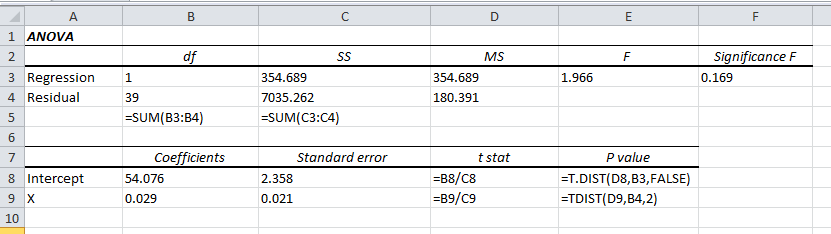a. Sample size = 1 + (Degree of freedom) = 1 + (1+39) = 41
b. Null and alternative hypotheses

H0: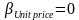H1: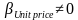The computed p value (0.175) is not lower than level of significance (0.05). Hence, insufficient witnesses are present to reject H0 and to accept H1 (Harmon, 2016). Thereby, slope is termed as insignificant and “unit price and supply is not correlated”.

c. R square (Coefficient of correlation)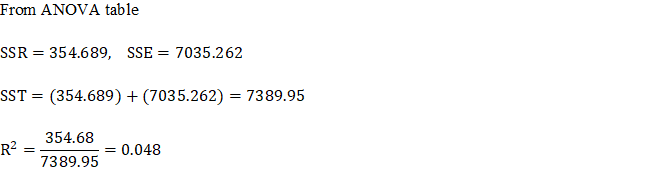The value of R square is quite less because only 4.8% changes in supply will be explained by changes in unit price of the product. Therefore, model is not termed as good fit.”

d. R (Coefficient of determination)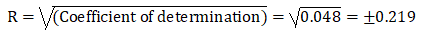The slope has positive (+ve) sign and thus, the applicable sign for R would also be positive. Hence, R would be +0.219 (Hair, et.al., 2016). The value is quite less (lower than 0.5) and thus, the association of the variable is low which is also evident from the hypothesis testing.”

e. The regression equation can be furnished from the regression model.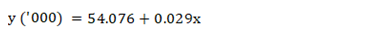The unit price is given as = \$50,000 and thus, x (‘000) = 50

Further,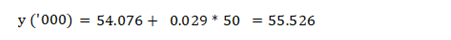The supply obtained is 55.526 thousands units or 55526 units.

### ANOVA Single Factor Test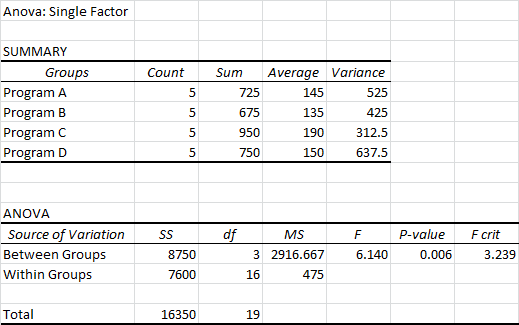Hypothesis testing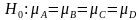(Means are equal for all four programs)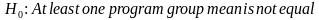.

Significance level =0.05 (Given)

Test statistic (F value) = 6.14

P value (From above) = 0.006

Observation: P value<< significance level (0.006 <0.05)

Result: Reject null hypothesis and accept alternative hypothesis

Conclusion: “At least one program group mean is not equal”

### A. Regression output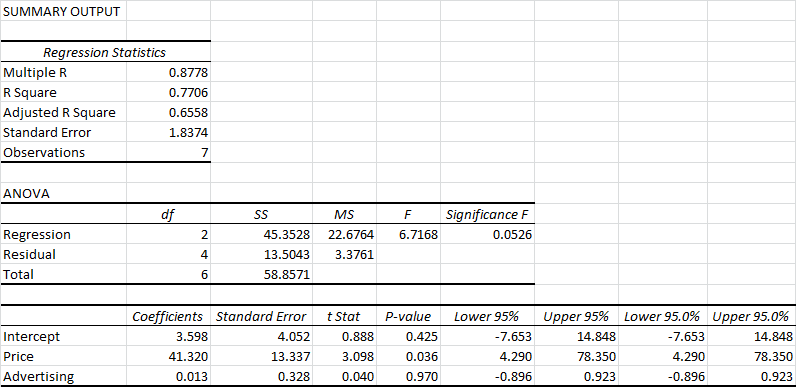### Equation of least square regression line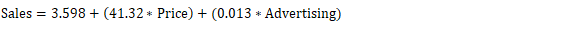b. Hypotheses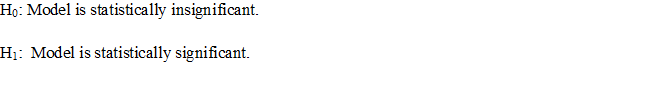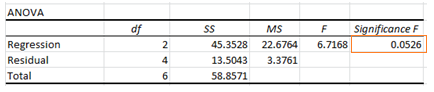Significance level = 0.10 (Given)

Test statistic (F) = 6.7168

Further, significance F = 0.0526

Observation: significance F << significance level (0.0526 <0.05)

Result: “Reject null hypothesis and accept alternative hypothesis (Hair, et.al., 2016)”

Conclusion: “Model is significant”

### c. Hypothesis testing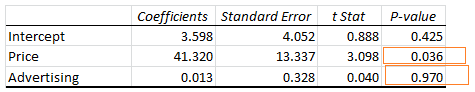Significance level = 0.10

For variable price: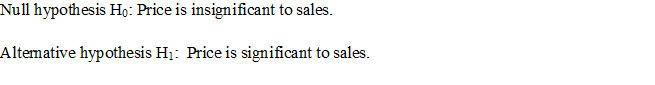Observation: p value << significance level (0.036 <0.10)

Result: “Reject null hypothesis and accept alternative hypothesis (Flick, 2015)

Conclusion: “Price is significant to sale”

### Observation: p value >> significance level (0.97 >0.10)

Result: Fails to reject null hypothesis and thus, cannot accept alternative hypothesis

Conclusion: “Advertising expenditure is not significant to sale”d. The new model will be produced by eliminating the advertising expenses from the mode.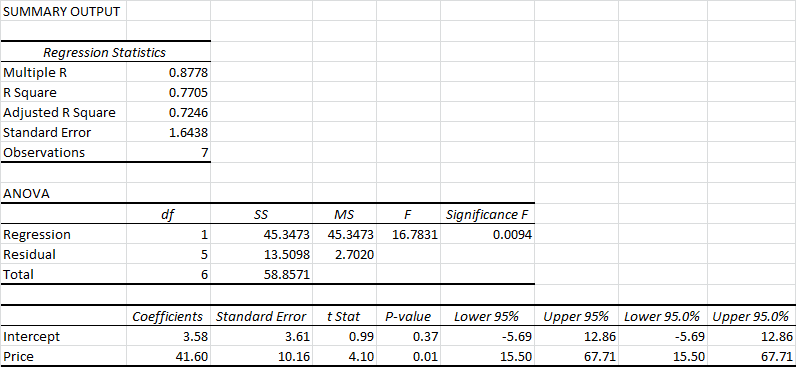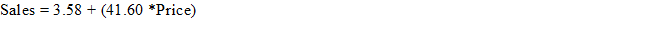### The equation of least square regression line

Slope indicates that when there is increase in price by \$1 then the corresponding sale would also increase by 41.60 units.”

#### Reference

1. Flick, U. (2015) Introducing research methodology: A beginner's guide to doing a research project. 4th ed. New York: Sage Publications.
2. Hair, J. F., Wolfinbarger, M., Money, A. H., Samouel, P., and Page, M. J. (2016) Essentials of business research methods. 2nd ed. New York: Routledge.
3. Harmon, M. (2016) Hypothesis Testing in Excel - The Excel Statistical Master. 7th ed. Florida: Mark Harmon.